## Selina Concise Chemistry Class 9 ICSE Solutions Atomic Structure and Chemical Bonding

APlusTopper.com provides step by step solutions for Selina Concise ICSE Solutions for Class 9 Chemistry Chapter 4 Atomic Structure and Chemical Bonding. You can download the Selina Concise Chemistry ICSE Solutions for Class 9 with Free PDF download option. Selina Publishers Concise Chemistry for Class 9 ICSE Solutions all questions are solved and explained by expert teachers as per ICSE board guidelines.

PAGE NO: 55

Question 1.
What is the contribution of the following in atomic structure?

2. Democritus

Solution:
Matter consisted of indestructible particles called paramanus (now called atoms).
A paramanu does not exist in the free state, rather it combines with other paramanus to form a bigger particle called the anu (now called a molecule).
Democritus called paramanu as atom which comes from the Greek word atomos, meaning indivisible.

Question 2.
State Dalton’s atomic theory.
Solution:
Main postulates of Dalton’s atomic theory:

• Matter consists of very small and indivisible particles called atoms.
• Atoms can neither be created nor be destroyed.
• The atoms of an element are alike in all respects, but they differ from the atoms of other elements.
• Atoms of an element combine in small numbers to form molecules.
• Atoms of one element combine with atoms of another element in a simple ratio to form molecules of compounds.
• Atoms are the smallest units of matter which can take part in a chemical reaction.

Question 3.
What is an α (alpha) particle?
Solution:
An α-particle is a doubly charged helium ion (He2+) containing two protons and two neutrons. It is formed by removing two electrons from the helium atom.

Question 4.
What are cathode rays? How are these rays formed?
Solution:
Cathode rays are the beam of electrons which travel from the negatively charged end (i.e. cathode to anode) of a vacuum tube, across a voltage difference between the electrodes placed at each end.
Formation of cathode rays:
Generally gases are poor conductors of electricity. However, when a high voltage charge from an induction coil is applied to tubes filled with gases at very low pressure (0.01 mm of mercury), the gases become good conductors of electricity and begin to flow in the form of rays.
These rays are called cathode rays and they travel from the cathode towards the anode.Question 5.
What is the nature of the charge on

1. Cathode rays and
2. Anode rays?

Solution:
Cathode rays are negatively charged as they consist of negatively charged particles called electrons.
Properties of cathode rays:

• They travel from the cathode to the anode in straight lines.
• They cause a greenish yellow fluorescence on a soda-glass screen placed in the tube.
• They are affected by an electric field, i.e. they are inflected towards the positive field and deflected from the negative field. This shows that they carry a negative charge.
• When a beam of cathode rays is made to fall upon hard metallic targets like tungsten, X rays are produced.
• They penetrate matter.
• They cause ionisation of the gas through which they pass.
• The ratio of the charge (s) to mass (m) of the particles constituting cathode rays remains the same (e/m = 1.76 × 1011 coulomb/kg) irrespective of the nature of the gas taken and of the metal forming the cathode.
• They produce the shadow of an opaque object placed in their path and make a light paddle wheel rotate.

Question 6.
How are X-rays produced?
Solution:
When a beam of cathode rays is made to fall upon hard metallic targets like tungsten, X-rays are produced.

Question 7.
Why are anode rays also called as ‘canal rays’?
Solution:
When perforated rays were used in the discharge tube, another set of rays travelling in a direction opposite to that of the cathode rays, i.e. from the anode towards the cathode, is seen, as in the figure below. These rays are called canal rays because they passed through the holes or canals in the cathode. These rays were named positive rays or anode rays.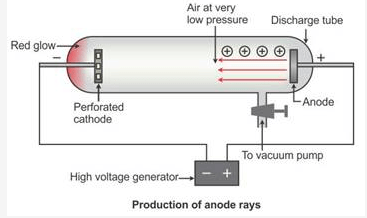Question 8.
How does cathode ray differ from as anode rays?
Solution:Question 9.
State one observation which shows that atom is not indivisible.
Solution: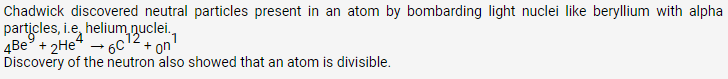Question 10.

1. Name an element which does not contain neutron
2. If an atom contains one electron and one proton, will it carry as a whole is neutral

Solution:

• Hydrogen does not contain any neutron.
• No. It is neutral.

Question 11.
On the basis of Thomson’s model of an atom explain how an atom as a whole is neutral.
Solution:
Thomson’s Atomic Model:
According to Thomson’s atomic model, an atom is made of positively charged substances in the form of a sphere. Electrons are embedded into this sphere, and the total positive charge of the sphere is equal to the total negative charge of electrons, and hence, the atom remained electrically neutral. This model was not accepted because it was unable to explain how positively charged particles were shielded from negatively charged particles without getting neutralised.Question 12.
Which sub-atomic particle was discovered by

1. Thomson
2. Goldstein

Solution:

• Thomson discovered particles.
• Goldstein discovered protons.

Question 13.
Name the sub-atomic particle whose charge is:

1. +1
2. -1
3. 0

Solution 13:

• Proton
• Electron
• Neutron

Solution 14:
Rutherford selected gold metal because he wanted as thin a layer as possible, and gold is the most malleable metal.
Heavy metals such as platinum will show the same observation with alpha particles as shown by gold foil.
If a light nucleus like lithium is used, then fast-moving alpha particles may even push the light nucleus aside and may not be deflected back.

Question 15.
On the basis of Rutherford’s model of an atom, which subatomic particle is present in the nucleus of an atom?
Solution:
According to Rutherford’s model of an atom, the subatomic particle in a nucleus is a proton.

Question 16.
Which part of atom was discovered by Rutherford?
Solution:
The nucleus was discovered by Rutherford.

Question 17.
How was it shown that atom has empty space?
Solution:
Rutherford performed an experiment by allowing a stream of alpha particles to pass through a very thin gold foil. He observed that alpha particles pass through the metal foil without deviating from their path. This shows that an atom contains a large empty space called nuclear space.

Question 18.
State one major drawback of Rutherford’s model
Solution:
One major drawback of Rutherford’s model was the comparison of electrons with the planets in the solar system.
Thus, when an electron moved around the nucleus continually, it should radiate energy, i.e. lose energy. As a result it should be gradually pulled towards the nucleus and end up colliding with it. This should result in the total collapse of the atom.
However, we know that the atom is structurally stable. Thus, Rutherford’s model could not explain this stability.

Question 19.
In the figure given alongside

1. Name the shells denoted by A,B, and C. Which shell has least energy
2. Name X and state the charge on it
3. The above sketch is of …………. Model of an atom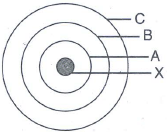Solution:
A is for K shell or I shell.
B is for L shell or II shell.
C is for M shell or III shell.
Shell K has the minimum amount of energy.
X is a nucleus; it is positively charged.
The above sketch is of Bohr model of an atom.

Question 20.
Give the postulates of Bohr’s atomic model
Solution:
Atom has a central nucleus surrounded by electrons.
The electrons revolve around the nucleus in definite circular paths called orbits.
Each orbit has fixed energy. Therefore, these orbits are also known as energy levels or energy shells.
These orbits or energy levels are represented either by a number 1, 2, 3,… known as the principal quantum number (n) of the orbit or by K, L, M,….
There is no change of energy of electrons as long as they keep revolving in the same energy level and the atom remains stable. Electrons can jump from the higher orbit to the lower orbit when they lose energy and jump to the next when they gain energy.

PAGE NO: 59

Question 1.

1. Name the three fundamental particles of an atom.
2. Give the symbol and charge of each particle.

Solution:
Symbol     Charge
Electron           e1-           -1
Proton             p1+         +1
Neutron            n              0
i. Electron 20
ii. Neutron 20
iii. Proton 20

Question 2.
Complete the table given below by identifying P, Q, R and S.Solution: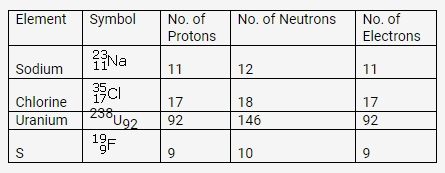Question 3.
The atom of an element is made up of 4 protons, 5 neutrons and 4 electrons. What are its atomic number and mass number?Solution:
Atomic number = Number of protons or number of electrons = 4
Mass number = Number of protons + Number of neutrons = 4 + 5 = 9

Question 4.
The atomic number and mass number of sodium are 11 and 23 respectively. What information is conveyed by this statement?Solution:
Atomic number (11) of sodium conveys information that the number of protons and electrons is the same.
Mass number (23) of sodium indicates the sum of protons and neutrons.

Question 5.
Write down the names of the particles represented by the following symbols and explain the meaning of superscript and subscript numbers attachedSolution:
p = proton
n = neutron
e = electron
Superscript number : These superscript number show their mass number
Subscript number : These numbers show their atomic number

Question 6.
From the symbol,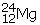state the mass number, the atomic number and electronic configuration of magnesium
Solution:
Mass number = 24
Atomic number = 12
No. of electrons = 24 – 12 = 12
Electronic configuration = 2, 8, 2

Question 7.
Sulphur has an atomic number 16 and a mass of 32.
State the number of protons and neutrons in the nucleus of sulphur. Give a simple diagram to show the arrangement of electrons in an atom of sulphur.
Solution:
Atomic number = 16
Atomic mass = 32
Number of protons = 16
Number of electrons = 16
Number of neutrons = 32 – 16 = 16
Electronic configuration = 2, 8, 6Question 8.
Explain the rule according to which electrons are filled in various energy levels.
Solution:
The maximum capacity of a shell to accommodate electrons is given by the general formula 2n2, where n is the serial number of a shell.
The maximum number of electrons possible in the outermost shell is 8 and that in the penultimate shell is 18.
It is not necessary for an orbit to be completed before another is formed. In fact, a new orbit is formed when the outermost shell attains 8 electrons.

Question 9.
Draw the orbital diagram of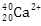ion and state the number of three fundamental particles present in it.
Solution:PAGE NO: 62

Question 1.
How does the Modern atomic theory contradict and correlate with Dalton’s atomic theory?
Solution:
The latest research on the atom has proved that most of the postulates of Dalton’s atomic theory contradict. However, Dalton was right that atoms take part in chemical reactions.
Comparisons of Dalton’s atomic theory with the modern atomic theory.

1. Dalton’s atomic theory:
• Atoms are indivisible.
• Atoms of the same element are similar in every respect.
• Atoms combine in a simple whole number ratio to form molecules.
• Atoms of different elements are different.
• Atoms can neither be created nor be destroyed.
2. Modern atomic theory:
• Atoms are no longer indivisible and consist of electrons, protons, neutrons and even more sub-particles.
• Atoms of the same element may differ from one another called isotopes.
• Atoms of different elements may be similar called isobars.
• Atoms combine in a ratio which is not a simple whole number ratio; e.g. in sugar, the C12H22O11 ratio is not a whole number ratio.

Question 2.

1. What are inert elements?
2. Why do they exist as monoatoms in molecules?
3. What are valence electrons?

Solution:
The elements have a complete outermost shell, i.e. 2 or 8 electrons. They ordinarily do not enter into any reaction.
These exist as monoatoms because molecules of these elements contain only one atom.
Valence electrons: The number of electrons present in the valence shell is known as valence electrons.

Question 3.
In what respects do the three isotopes of hydrogen differ? Give their structures.
Solution:
The three isotopes differ only due to their mass number which is respectively 1, 2 and 3 and named protium, deuterium and tritium.Question 4.
Match the atomic numbers 4,14,8,15 and 19 with each of the following:

1. A solid non-metal of valency 3.
2. A gas of valency 2.
3. A metal of valency 1.
4. A non-metal of valency 4.

Solution:Question 5.
Draw diagrams representing the atomic structures of the following:

1. Sodium atom
2. Chlorine ion
3. Carbon atom
4. Oxygen ion

Solution: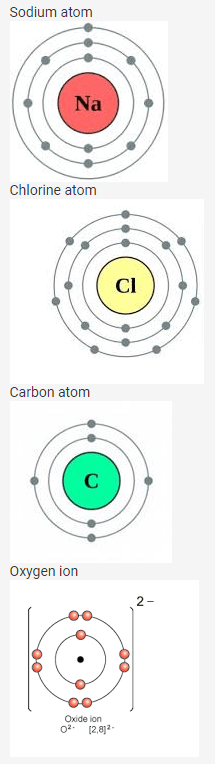Question 6.
What is the significance of the number of protons found in the atoms of different elements?
Solution:
The number of protons in the nucleus of an atom determines the element type of the atom.

Question 7.
Elements X, Y and Z have atomic numbers 6,9 and 12 respectively. Which one:
(a) Forms an onion
(b) Forms a cation
(c) Has four electrons in its valence shell?
Solution:
Atomic numbers of
X 6 (2, 4)
Y 9 (2, 7)
Z 12 (2, 8, 2)
Y(2, 7) forms an anion.
Z(2, 8, 2) forms a cation.
X(2, 4) has four electrons in the valence shell.

Question 8.
Element X has electronic configuration 2,8,18,8,1. Without identifying X,

1. Predict the sign and charge on a simple ion of X.
2. Write if X will be an oxidizing agent or a reducing agent. Why?

Solution:
X1+
Oxidising agent, because it has the ability to donate electrons.

Question 9.
Define the terms:

1. Mass number
2. Ion
3. Cation
4. Anion
5. Element
6. orbit

Solution:
Mass number is the sum of the number of protons and the number of neutrons in the nucleus of an atom.
Ion is an atom or molecule which carries a positive or negative charge because of loss or gain of electrons.
Cation is a positively charged ion which is formed when an atom loses one or more electrons; for example, Na+, Hg2+ and Ca2+.
Anion is a negatively charged ion which is formed when an atom gains one or more electrons; for example, Cl and I.
Element is a substance which cannot be split up into two or more simple substances by usual chemical methods of applying heat, light or electric energy; for example, hydrogen, oxygen and chlorine.
Orbit is a circular path around the nucleus in which electrons of the atom revolve.

Question 10.
From the symbol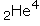for the element helium, write down the mass number and the atomic number of the element
Solution:
Atomic number = 2
Mass number = 4

Question 11.
Five atoms are labeled A to E(a) Which one of these atoms:
i. contains 7 protons
ii. has electronic configuration 2,7
(b) Write down the formula in the compound formed between C and D
Predict : (i) metals (ii) non-metals

Solution:

• Atom E contains 7 protons.
Atom B has an electronic configuration 2, 7.
• Atom C stands for 7Li3, Atom D stands for 8O16.
Hence, compound formula is Li2O.
• Metals: A and C, Non-metals: B, D, E

Question 12.
An atom of an element has two electrons in the M shell.
What is the (a) atomic number (b) number of protons in this element?
Solution:
Number of electrons in the M shell = 2
So, the number of electrons in the K and L shells will be = 2, 8
Hence, atomic number = 2 + 8 + 2 = 12
Number of protons = 12

PAGE NO : 63

Question 13Solution:Question 14.
What are nucleons? How many nucleons are present in phosphorus? Draw its structure.
Solution:
Nucleons: Particles which constitute the nucleus are called nucleons.
Protons and neutrons are the nucleons.
Atomic mass of phosphorus = 31
Atomic number = 15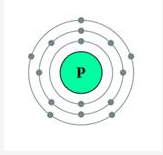Question 15.
What are isotopes? With reference to which fundamental particle do isotopes differ? Give two uses of isotopes.
Solution:
Isotopes: Atoms of the same element having the same atomic number but different mass numbers.
Isotopes differ with reference to neutrons.
Uses of isotopes:
Some isotopes are radioactive, i.e. isotopes of cobalt are used for treating cancer and other diseases.
An isotope of 235U is used as a fuel in a nuclear reactor.

Question 16.
Why dohave the same chemical properties? In what respect do these atoms differ?
Solution: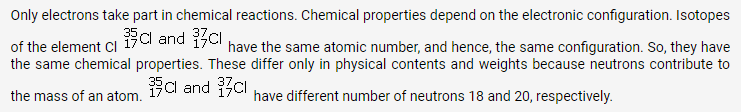Question 17.
Explain fractional atomic mass. What is the fractional mass of chlorine?
|Solution:
Atomic masses of the isotopes of chlorine are 35 and 37. However, in any given sample of chlorine gas, the isotopes occur in the approximate ratio 3:1, 75% of Cl35 and 25% of Cl37. So, the relative atomic mass or atomic weight of chlorine is 35.5.
Fractional atomic mass of chlorine
At. Mass = (3(35) + 1(37)) / 2 = (105 + 37) / 2

Question 18.
(a) What is meant by ‘atomic number of an element”?
(b) Complete the table given below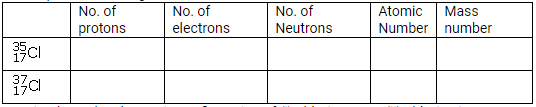(c) Write down the electronic configuration of (i) chlorine atom (ii) chlorine ion
Solution: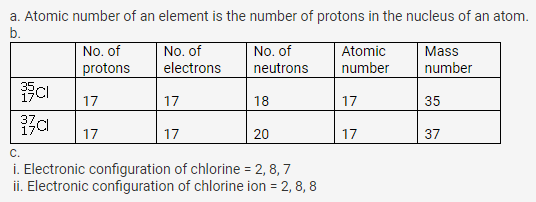Question 19.
Name the following:

1. The element which does not contain any neutron in its nucleus.
2. An element having valency ‘zero’
3. Metal with valency 2
4. Two atoms having the same number of protons and electrons but different number of neutrons.
5. The shell closest to the nucleus of an atom

Solution:
Hydrogen
Helium
Magnesium
Hydrogen and carbon
K

Question 20.
Give reasons
(a) Physical properties of isotopes are different.
(b) Argon does not react.
(c) Actual atomic mass is greater than mass number.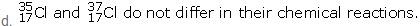Solution:
Physical properties depend on atomic mass, and isotopes have different mass number, i.e. they have different number of neutrons. So, isotopes have different physical properties.
Argon does not react as it has the outermost orbit complete, i.e. 8 electrons in the outermost shell.
Actual atomic mass is greater than the mass number because the mass number is a whole number approximation of atomic mass unit. In fact, neutrons are slightly heavier than protons, and an atom has over 200 sub-atomic particles.
are isotopes of chlorine element which differ in the number of neutrons, whereas chemical properties are determined by the electronic configuration of an atom. Isotopes of an element are chemically alike.

Question 21.
An element A atomic number 7 mass numbers 14
B electronic configuration 2,8,8
C electrons 13, neutrons 14
D Protons 18 neutrons 22
E Electronic configuration 2,8,8,1
State (i) Valency of each element (ii) which one is a metal (iii) which is non-metal (iv) which is an inert gas
Solution:

• Element A
Atomic number = 7 = Number of electrons = 2, 5
Valency of A = 8 – 5 = 3
Element B
Electronic configuration 2, 8, 8
Valency of B = Zero
Element C has 13 electrons
Electronic configuration = 2, 8, 3
Valency of C = 3
Element D
Protons = 18 = Electrons = 2, 8, 8
Valency of D = Zero
Element E
Electronic configuration = 2, 8, 8, 1
Valency of E = 1
• C and E are metals.
• A is a non-metal.
• A, C and E are not inert gases.

Question 22.
Choose the correct option
(a) Rutherford’s alpha-particle scattering experiment discovered

1. Electron
2. Proton
3. Atomic nucleus
4. Neutron

(b) Number of valence electrons in O2- is :

1. 6
2.  8
3. 10
4. 4

(c) Which of the following is the correct electronic configuration of potassium?

1. 2,8,9
2. 8,2,9
3. 2,8,8,1
4. 1,2,8,8

Solution:
Atomic nucleus

[2, 8, 8, 1]

Question 23.
Explain

1. Octet rule for formation of sodium chloride
2. Duplet rule for formation of hydrogen

Solution:
Elements tend to combine with one another to attain the stable electronic configuration of the nearest inert gas.

• Sodium chloride
Sodium atom has 1 electron in the valence shell which it donates to the chlorine atom with 7 electrons in the valence shell to attain the stable electronic configuration of the nearest inert gas, i.e. 8 electrons in the valence shell. This is known as the octet rule. These elements combine to form sodium chloride.
• Hydrogen
Hydrogen atom has one electron in the valence shell which it shares with another hydrogen atom having one electron to complete its duplet state, i.e. two electrons in the valence shell and resulting in the formation of hydrogen.

Question 24.
Complete the following table relating to the atomic structure of some elements.Solution: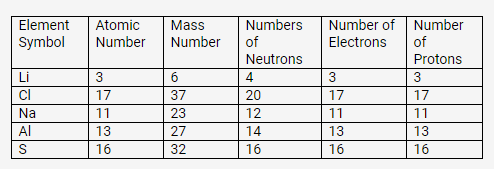PAGE NO: 67

Question 1.
How do atoms attain noble gas configurations?
Solution:
Combining atoms attain the noble gas configuration by transferring or sharing electrons.

Question 2.
Define electrovalent bond.
Solution:
A chemical bond may be defined as the force of attraction between any two atoms in a molecule to maintain stability.
The chemical bond formed due to the electrostatic force of attraction between a cation and an anion is called an electrovalent bond.
The chemical bond formed between two combining atoms by mutual sharing of one or more pairs of electrons is called a covalent bond.

Question 3.
Elements are classified as metals, non-metal, metalloids and inert gases. Which of them form electrovalent bond?
Solution:
Conditions for the formation of an ionic bond:

• Ionisation potential (IP)
Lower the value of IP of a metallic atom, greater the ease of formation of the cation.
• Electron affinity
Higher the value of EA of a non-metallic atom, greater the ease of formation of the anion.
• Electronegativity
Larger the electronegativity difference between combining atoms, greater the ease of electron transfer.

Question 4.
(a) An atom X has three electrons more than the noble gas configuration. What type of ion will it form?
(b) Write the formula of its (X)

1. sulphate
2. nitrate
3.  iii. phosphate
4. carbonate
5. hydroxide.

Solution:
It will form a positive ion, i.e. cation.

• X(SO4)3
• X(NO3)3
• XPO4
• X2(CO3)3
• X(OH)3

Question 5.
Mention the basic tendency of an atom which makes it to combine with other atoms
Solution:
Besides chaos, everything in this world wants stability. The same is the case with atoms. For atoms, stability means having the electron arrangement of an inert gas, i.e. octet in the outermost shell. Helium has two electrons (duplet), while all other inert gases, i.e. neon, argon, krypton, xenon and radon have eight electrons (octet) in the outermost shell.

Question 6.
What type of compounds are usually formed between metals and non-metals and why?
Solution:
Electrovalent compounds are usually formed between metals and non-metals.
Atoms of metallic elements which have 1, 2 or 3 valence electrons can lose electron(s) to atoms of non-metallic elements which have 5, 6 or 7 valence electrons and thereby form an electrovalent bond.

Question 7.

1. In the formation of the compound XY2, an atom X gives one electron to each Y atom. What is the nature of bond in XY2?
2. Draw the electron dot structure of this compound.

Solution:

1. Nature of bond in XY2 is an ionic bond.
2. Electron dot structure of XY2:Question 8.
An atom X has 2, 8, 7 electrons in its shell. It combines with Y having 1 electron in its outermost shell.

1. What type of bond will be formed between X and Y?
2. Write the formula of the compound formed.

Solution:
Ionic bond
XY

Question 9.
Draw orbit structure diagram of sodium chloride (NaCl) and calcium oxide (CaO).
Solution: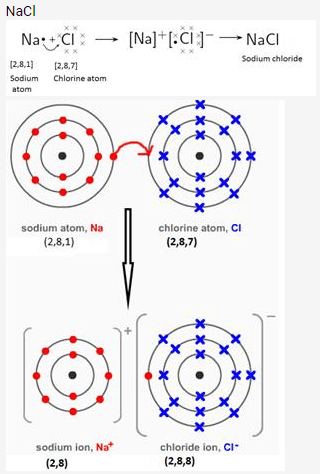Question 10.
Compare :

(a) Sodium atom and sodium ion

(b) Chlorine atom and chloride ion, with respect to

1.  Atomic structure
2. Electrical state

Solution 10: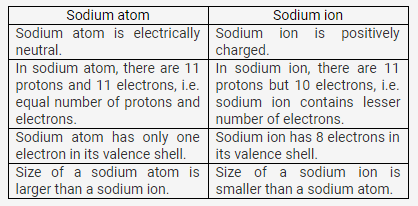In chlorine atom, the number of protons (17) is equal to the number of electrons (17).
In chloride ion, there are 17 protons but 18 electrons.
Chlorine atom is electrically neutral. Chloride ion is negatively charged.
Chlorine atom is reactive. It reacts with sodium vigorously forming sodium chloride. Chloride ion is unreactive. It does not react with sodium.
Chlorine (Cl2) is a poisonous, toxic, corrosive gas which is used in the manufacture of bleaching agents and disinfectants. It is non-toxic and readily adsorbed by plants.

Question 11.
The electronic configuration of fluoride ion is the same as that of neon atom. What is the difference between the two?
Solution:
Fluoride ion is a negatively charged ion with 9 protons and 10 electrons.
Neon atom is electrically neutral with 10 protons and 10 electrons.

Question 12.
1. What do you understand by redox reactions?
2. Explain oxidation and reduction in terms of loss or gain of electrons.

Solution 12:
Transfer of electron(s) is involved in the formation of an electrovalent bond. The electropositive atom undergoes oxidation, while the electronegative atom undergoes reduction. This is known as a redox process.
Oxidation: In the electronic concept, oxidation is a process in which an atom or ion loses electron(s).
Zn → Zn2+ + 2e
Reduction: In the electronic concept, reduction is a process in which an atom or ion accepts electron(s).
Cu2+ + 2e → Cu

• Zn → Zn2++ 2e (Oxidation)
Pb2+ + 2e → Pb (Reduction)
• Zn → Zn2+ + 2e (Oxidation)
Cu2+ + 2e→ Cu (Reduction)
• Cl+ 2e →2Cl (Reduction)
2Br → Br2 + 2e (Oxidation)
• Sn2+ → Sn4+ + 2e (Oxidation)
2Hg2+ + 2e → Hg2 (Reduction)
• Cu+ → Cu2+ + e (Oxidation)
Cu+ + e → Cu (Reduction)
Question 13.
Potassium (at No.19) and chlorine (at No.17) react to form a compound. Explain on the basis of electronic concept:
i. Oxidation
ii. Reduction
iii. Oxidizing agent
iv. Reducing agent

2K + Cl2 → 2KCl

• Oxidation: In the electronic concept, oxidation is a process in which an atom or ion loses electron(s).
K → K+ + e
• Reduction: In the electronic concept, reduction is a process in which an atom or ion accepts electron(s).
Cl2 + 2e→ 2Cl
• Oxidising agent
An oxidising agent oxidises other substances either by accepting electrons or by providing oxygen or an electronegative ion, or by removing hydrogen or an electropositive ion.
Cl2 + 2e→ 2Cl
• Reducing agent
A reducing agent reduces other substances either by providing electrons or by providing hydrogen or an electropositive ion, or by removing oxygen or an electronegative ion.
K → K+ + e

PAGE NO: 73

Solution 1:
They are good conductors of electricity in the fused or aqueous state because electrostatic forces of attraction between ions in the solid state are very strong and these forces weaken in the fused state or in the solution state. Hence, ions become mobile.
In electrovalent compounds, there exists a strong force of attraction between the oppositely charged ions, so a large amount of energy is required to break the strong bonding force between ions. So, they have high boiling and melting points.
In covalent compounds, they have weak forces of attraction between the binding molecules, thus less energy is required to break the force of binding. So, they have low boiling and melting points.
As water is a polar compound, it decreases the electrostatic forces of attraction, resulting in free ions in aqueous solution. Hence, electrovalent compounds dissolve.
Covalent compounds do not dissolve in water but dissolve in organic solvents. Organic solvents are non-polar; hence, these dissolve in non-polar covalent compounds.
Electrovalent compounds are usually hard crystals yet brittle because they have strong electrostatic forces of attraction between their ions which cannot be separated easily.
Polar covalent compounds conduct electricity because they form ions in their solutions.

Solution 2:
The nature of the solid is ionic.
Ionic compounds are hard crystalline solids with high melting points because of a strong force of attraction between the oppositely charged ions. So, a large amount of energy is required to break the strong bonding force between ions.
Electrovalent compounds are soluble in water. As water is a polar compound, it decreases the electrostatic forces of attraction, resulting in free ions in aqueous solution. Hence, they dissolve.

Question 3
Match the atomic numbers 4,8,10,15 and 19 with each of the following:

1. Element which can form trivalent ion
2. Element with four shells
3. Element with 6 valence electrons
4. Element which does not form ion

Solution:
15
8
19
10

Question 4.
(a) Elements X,Y and Z have atomic numbers 6,9 and 12 respectively. Which one:

1. Forms an anion
2. Forms a cation

(b) State the type of bond between Y and Z and give its molecular formula.
Solution:
i. Y = 9
ii. Z = 12
Ionic bond with molecular formula ZY2.

Question 5.
Taking MgCl2 as an electrovalent compound, CCl4 as a covalent compound, give four difference between electrovalent and covalent compounds
Solution: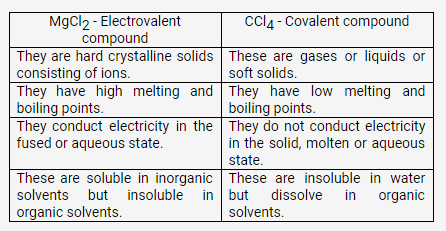Question 6.
Potassium chloride is an electrovalent compound, while hydrogen chloride is covalent compound, But, both conduct electricity in their aqueous solutions. Explain.
Solution:
Potassium chloride is an electrovalent compound and conducts electricity in the molten or aqueous state because the electrostatic forces of attraction weaken in the fused state or in aqueous solution.
Polar covalent compounds like hydrogen chloride ionise in their solutions and can act as an electrolyte. So, both can conduct electricity in their aqueous solutions.

Question 7.
Name two compounds that are covalent when taken pure but produce ions when dissolved in water.
Solution:
HCl and NH3
HCl + H2O → H3O+ + Cl
NH3 + H2O →NH4+ + OH

Question 8.
An element M burns in oxygen to form an ionic compound MO. Write the formula of the compounds formed if this element is made to continue with chlorine and sulphur separately.
Solution:
Formula of compound when combined with sulphur – MS
Formula of compound when combined with chlorine – MCl2

Solution 9:

Question 10.
State the type of bonding in the following molecules.

1. Water,
2. Calcium oxide
3. Hydrogen chloride

Solution:
Polar covalent bond
Ionic bond
Polar covalent bond

Question 11.
Element M forms a chloride with the formula MCl2 which is a solid with high melting point. What type of bond is in MCl2 . Write the formula of the compound when M combine with sulphur, oxygen and nitrogen.
Solution:
The bond formed between metal and nonmetal is ionic bond.
Bond formed between metal M and chlorine is ionic bond.
When metal M combines with sulphur – MgS
When metal M combines with  oxygen – MgO
(b) Mg

Solution 12:
C. Covalent
C. Low melting point and low boiling point
D. Not conduct electricity
Y is getting reduced.
Anode

Solution 13:
B. It has a high melting point.
A. It loses electrons and is oxidised.

Question 14.
Identify the following reactions as either oxidation or reduction:

1. O + 2e → O2-
2. K – e → K+
3. Fe3+ + e → Fe2+
4. Zn → Zn2+ + 2e-

Solution:
Reduction
Oxidation
Reduction

Question 15.

1. Name the charged particles which attract one another to form electrovalent compounds.
2. In the formation of electrovalent compounds, electrons are transferred from one element to another. How are electrons involved in the formation of a covalent compound?
3. The electronic configuration of nitrogen is (2, 5). How many electrons in the outer shell of a nitrogen atom are not involved in the formation of a nitrogen molecule?
4. In the formation of magnesium chloride (by direct combination between magnesium and chlorine), name the substance that is oxidized and the substance that is reduced.

Solution:
Cation and anion
By mutual sharing of electrons
Two
Magnesium is oxidised and chlorine is reduced.

PAGE NO : 74
Solution 16:
C. Consists of oppositely charged ions
Covalent bond

More Resources for Selina Concise Class 9 ICSE Solutions